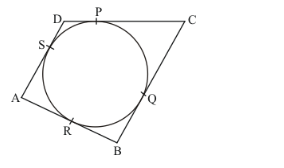# The length of three concesutive sides of a quadrilateral circumscribing a circle

Question:

The length of three concesutive sides of a quadrilateral circumscribing a circle are 4 cm, 5 cm, and 7 cm respectively. Determine the length of the fourth side.

Solution:

Let us first put the given data in the form of a diagram.From the property of tangents we know that the length of two tangents drawn from the same external point will be equal. Therefore we have,

AR = SA

Let us represent AR and SA by ‘a’.

Similarly,

QB = RB

Let us represent SD and DP by ‘b’

PC = CQ

Let us represent PC and PQ by ‘c’

SD = DP

Let us represent QB and RB by ‘d

It is given that,

AB = 4

AR + RB =4

a + b = 4

b = 4 − a …… (1)

Similarly,

BC = 5

That is,

b + c = 5

Let us substitute for b from equation (1). We get,

4 − a + c = 5

c − a = 1

c = a + 1 …… (2)

CD = 7

c + d = 7

Let us substitute for c from equation (2). We get,

a + 1 + d = 7

a + d = 6

In the previous section we had represented AS and SR with ‘a’ and SD and DP with ‘b’. We shall now put AS in place of ‘a’ and SD in place of ‘d’. We get,

AS + SD = 6